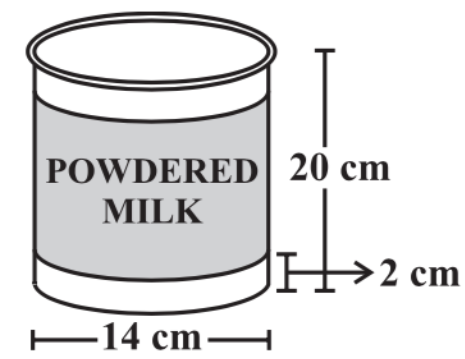# A company packages its milk powder in a cylindrical container whose base has a diameter of $14\text{ cm}$ and height $\text{20 cm}$. The company places a label around the surface of the container (as shown in the figure). If the label is placed $\text{2 cm}$ from top and bottom, what is the area of the label?Verified
204.9k+ views
Hint: As the label is also cylindrical in shape so the area of the label is equal to the surface area of the cylinder which is equal to $2\pi rh$ as height and diameter are given in the question. We will calculate the radius by using the relation $\text{Radius=}\dfrac{Diameter}{2}$ and substitute the values in the formula to obtain the desired result.

Complete step-by-step solution:
We have given that a label is placed on the surface of a cylindrical container whose base has a diameter of $14\text{ cm}$ and height $\text{20 cm}$. The label is placed $\text{2 cm}$ from top and bottom.
We have to find the area of the label.
We have given that the diameter of the container is $14\text{ cm}$.
We know that $\text{Radius=}\dfrac{Diameter}{2}$, so the radius will be $\dfrac{14\text{ cm}}{2}=7\text{ cm}$
We have given that the height of the container is $\text{20 cm}$ and the label is placed $\text{2 cm}$ from top and bottom, so the height of the label will be $20-\left( 2+2 \right)=16\text{ cm}$
Now, the label is also cylindrical in shape so the area of the label is equal to the surface area of the cylinder, which is given by $2\pi rh$.
Substituting the values, we get
\begin{align} & \text{Area of label =}2\pi rh \\ & \text{Area of label =}2\times \dfrac{22}{7}\times 7\times 16 \\ & \text{Area of label =44}\times \text{16} \\ & \text{Area of label =704 c}{{\text{m}}^{2}} \\ \end{align}
So, the area of label is $\text{704 c}{{\text{m}}^{2}}$.

Note: The key concept to solve this question is the area of the cylinder. Here in this question we only consider the surface area of the cylinder because the label is placed only on the surface of the container. When we use the height of the label we have to subtract the height $4\text{ cm}$ from the height of the cylinder because the label is placed $\text{2 cm}$ from both top and bottom.